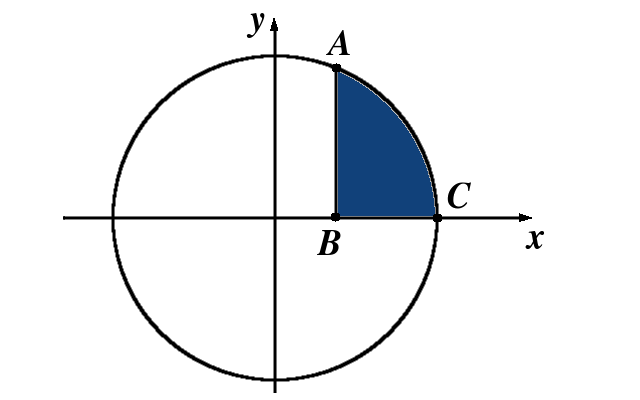# Integration by Parts

## Introduction

Finding the indefinite integral of the function $$(x\cos x+\sin x)$$ is difficult on the first sight. However, one hint changes the world:

You may now try to solve the problem yourself, and then look at the following example that solves the problem.

Example 1 Find $$\int \left(x\cos x+\sin x\right)\,dx$$.

The following problems are similar in nature, and it is a good idea to work on them before proceeding to the theory of integration by parts.

Example 2 Find $$\int \left(x^2\cos x+2x\sin x\right)\,dx$$.

Example 3 Find $$\int \left(x^2+2x\right)e^x\,dx$$.

We may now solve a problem similar to the one from Example 1:

Example 4 Find $$\int x\cos x\,dx$$.

## The method of integration by parts

Theorem (Integration by parts)

If $$u$$ and $$v$$ are differentiable functions then

$\int u(x)\cdot v^{\prime}(x)\,dx=u(x)\cdot v(x)-\int v(x)\cdot u^{\prime}(x)\,dx.$

Let us color the integral on the left in blue, and the integral on the right in green.

$$\int u(x)\cdot v^{\prime}(x)\,dx$$$$= u(x)\cdot v(x)-$$ $$\int v(x)\cdot u^{\prime}(x)\,dx$$.

The idea of the integration by parts is to start with difficult blue integral, and end up with an easy green integral. In Example 4, the integral $$\int x\cos x\,dx$$ was blue, and $$\int \sin x\,dx$$ was green.

Example 5 Find $$\int x e^x\,dx$$.

Example 6 Find $$\int x \sin x\,dx$$.

Example 7 Find $$\int \ln x\,dx$$.

Example 8 Find $$\int \sin x\cdot e^x\,dx$$.

## Practice problems

Problem 1. Determine the indefinite integral $\int x\arctan x\,dx.$

Problem 2. Determine the integral $\int_0^{\frac{\pi}2} x\sin x\,dx.$

Problem 3. Determine the indefinite integral $\int_1^5 x\ln x\,dx.$

Problem 4. Find the integral $\int_{-5}^5 x^4\cdot \sin\left(x^3\right)\,dx.$

Problem 5. Let $$k$$ be the circle of radius $$6$$ with center at the origin. Let $$A(3,4)$$, $$B(3,0)$$, and $$C(5,0)$$ be three points such that $$A$$ and $$C$$ are on the circle and $$B$$ is the projection of $$A$$ to the $$x$$ axis. Find the area of the figure bounded by the segments $$AB$$ and $$BC$$, and the arc $$\stackrel \frown{AC}$$ of the circle $$k$$.